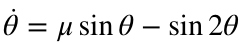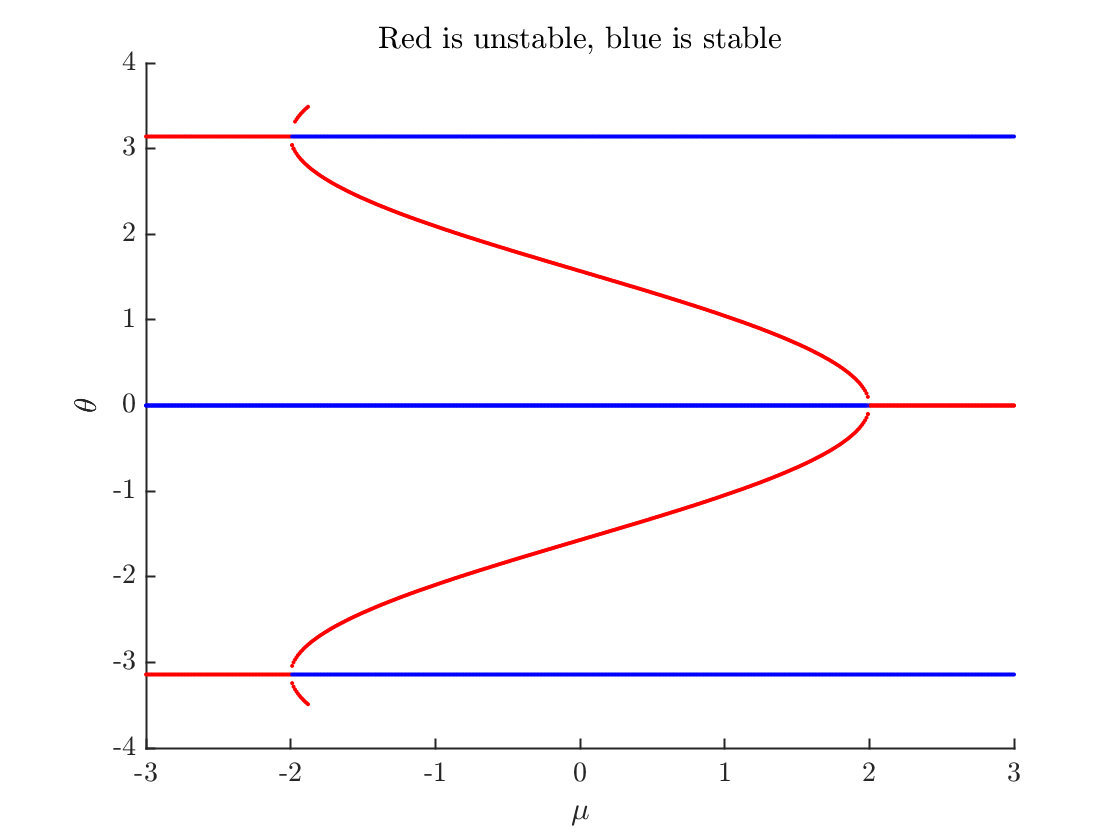# Strogatz Problem 4.3.3

## Math 473, Fall 2020

This is a small demonstration program that allows you to draw a simple bifurcation diagram. There are much better (and more mathematically sophisticated) ways to draw a bifurcation diagram than this quick-and-dirty code. Those are called continuation methods and there is a great continuation package for MATLAB called matcont.
Let's draw a bifurcation diagram fortheta=linspace(-3.5,3.5,101);
f=@(theta,mu) mu*sin(theta)-sin(2*theta);
fprime=@(theta,mu) mu*cos(theta)-2*cos(2*theta);
nMu=601;
muVector=linspace(-3,3,nMu);
simpleBifurcationDiagram(f,fprime,theta,muVector);
xlabel('$\mu$')
ylabel('$\theta$')
title('Red is unstable, blue is stable')## Subroutines section

function simpleBifurcationDiagram(f,fprime,xVector,muVector)
clf; hold on;
nMu=length(muVector);
for k=1:nMu
mu=muVector(k);
fmu=@(theta)f(theta,mu);
fVector=f(xVector,mu);
[m,p]=signChanges(fVector);
n=length(m);
for j=1:n
Theta=fzero(fmu,xVector(p(j)));
if fprime(Theta,mu)>0
plot(mu,Theta,'r.')
else
plot(mu,Theta,'b.')
end
end
end
end

#### A small function to detect sign changes

function [m,p]=signChanges(y)
s=y(1:end-1).*y(2:end); % <=0 if or only if a sign change or a zero
[m,p]=find(s<=0);
end